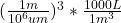## A vessel is filled at a rate of 3.41 cubic micrometers per minute. If the vessel has a volume of 54 liters, how many seconds will it t

Question

A vessel is filled at a rate of 3.41 cubic micrometers per minute. If the vessel has a volume of 54 liters, how many
seconds will it take to fill the vessel? provide a step buy step explanation.

in progress 0
7 months 2021-07-12T10:12:08+00:00 1 Answers 32 views 0

## Answers ( )

9.5×10¹⁷ s

Explanation:

First we convert 3.41 cubic micrometers (um³) to liters (L), as such:

• 3.41 um³ *= 3.41×10⁻¹⁵ L

With the converted rate of 3.41×10⁻¹⁵ L/min, we can calculate how many minutes it would take to fill a 54 L vessel:

• 54 L ÷ 3.41×10⁻¹⁵ L/min = 1.58×10¹⁶ min

Finally we convert 1.58×10¹⁶ minutes to seconds:

• 1.58×10¹⁶ * 60 = 9.5×10¹⁷ s